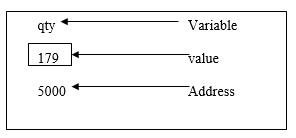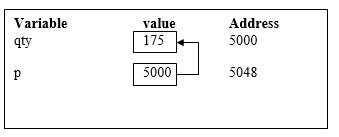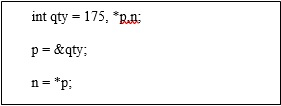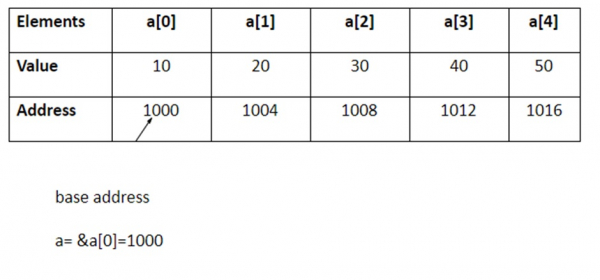# Explain pointers and one-dimensional array in C language

Pointer is a variable that stores the address of another variable.

## Features

The features of pointer are explained below −

• Pointer saves the memory space.
• Execution time of pointer is faster because of direct access to the memory location.
• With the help of pointers, the memory is accessed efficiently, i.e., memory is allocated and deallocated dynamically.
• Pointers are used with data structures.

## Pointer declaration, initialization and accessing

Consider the following statement −

int qty = 179;

In memory, the variable can be represented as follows −### Declaring a pointer

It means ‘p’ is a pointer variable which holds the address of another integer variable, as mentioned in the statement below −

Int *p;

### Initialization of a pointer

Address operator (&) is used to initialise a pointer variable.

For example −

int qty = 175;
int *p;
p= &qty;Accessing a variable through its pointer

To access the value of a variable, indirection operator (*) is used.

For example −Here, ‘*’ can be treated as value at address.

The two statements are equivalent by the following statement −

p = &qty;
n = *p; n =qty

## Pointers and one-dimensional arrays

• The compiler allocates Continuous memory locations for all the elements of the array.
• The base address = first element address (index 0) of the array.
• For Example − int a  = {10, 20,30,40,50};

### Elements

The five elements are stored as follows −• If ‘p’ is declared as integer pointer, then, an array ‘a’ can be pointed by the following assignment −

p = a;
(or) p = &a;
• Every value of 'a' is accessed by using p++ to move from one element to another element. When a pointer is incremented, its value is increased by the size of the datatype that it points to. This length is called the "scale factor".

• The relationship between ‘p’ and 'a' is explained below −

P = &a = 1000
P+1 = &a = 1004
P+2 = &a = 1008
P+3 = &a = 1012
P+4 = &a = 1016
• Address of an element is calculated using its index and the scale factor of the datatype. An example to explain this is given herewith.

Address of a = base address + (3* scale factor of int)
= 1000 + (3*4)
= 1000 +12
= 1012
• Pointers can be used to access array elements instead of using array indexing.

• *(p+3) gives the value of a.

a[i] = *(p+i)

## Example program

Following is the C program for pointers and one-dimensional arrays −

Live Demo

#include<stdio.h>
main ( ){
int a;
int *p,i;
printf ("Enter 5 lements");
for (i=0; i<5; i++)
scanf ("%d", &a[i]);
p = &a;
printf ("Elements of the array are");
for (i=0; i<5; i++)
printf("%d", *(p+i));
}

## Output

When the above program is executed, it produces the following result −

Enter 5 elements : 10 20 30 40 50
Elements of the array are : 10 20 30 40 50